# Class 12 Solid State NCERT Solutions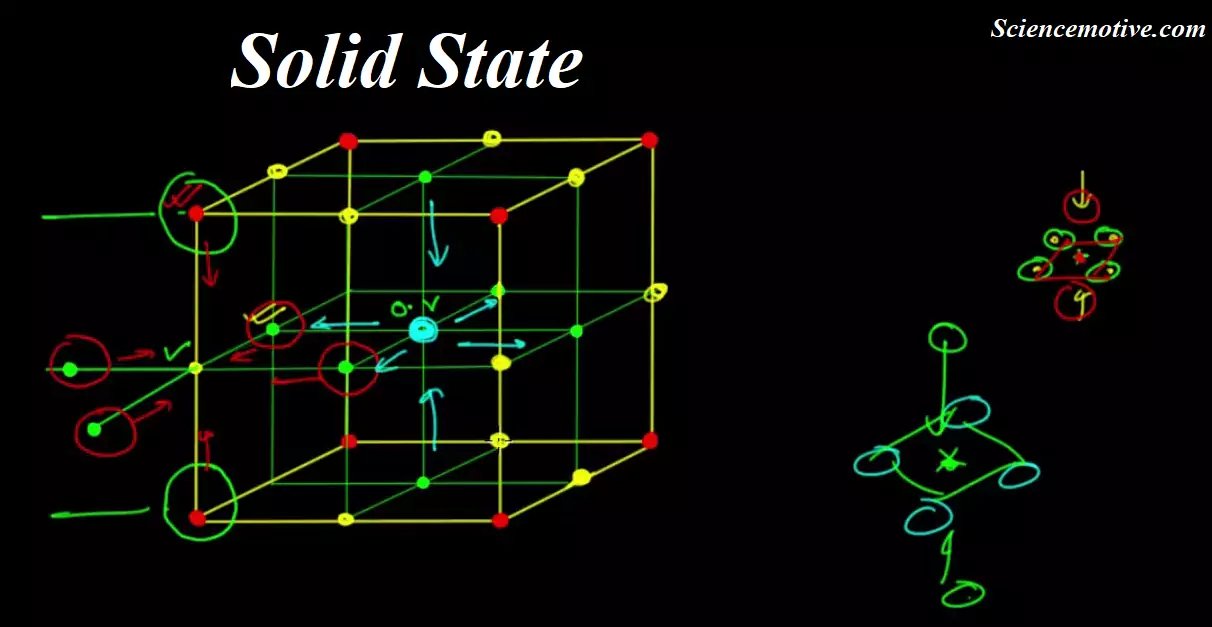Class 12 Solid State NCERT Solutions

Intext Solutions
The Solid State

Que 1.1: Why are solids rigid?
Ans. The intermolecular forces of attraction that are present in solids are very strong. The constituent particles of solids cannot move from their positions i.e., they have fixed positions. However, they can oscillate about their mean positions. This is the reason solids are rigid.

Que 1.2: Why do solids have a definite volume?
Ans. The intermolecular forces of attraction that are present in solids are very strong. The constituent particles of solids have fixed positions i.e., they are rigid. Hence, solids have a definite volume.

Que 1.3: Classify the following as amorphous or crystalline solids:
Polyurethane, naphthalene, benzoic acid, Teflon, potassium nitrate, cellophane, polyvinyl chloride, fibreglass, copper.
Ans 1.3. Amorphous solids: Polyurethane, Teflon, cellophane, polyvinyl chloride, fibreglass Crystalline solids: Naphthalene, benzoic acid, potassium nitrate, copper

Que 1.4: Why is glass considered a supercooled liquid?
Ans 1.4. Similar to liquids, glass has a tendency to flow, though very slowly. Therefore, glass is considered a supercooled liquid. This is the reason that glass windows and doors are slightly thicker at the bottom than at the top.

Que 1.5: Refractive index of a solid is observed to have the same value along with all directions. Comment on the nature of this solid. Would it show a cleavage property?
Ans 1.5: Anisotropic solid has the same value of physical properties when measured along with different directions. Therefore, the given solid, having the same value of refractive index along with all directions, is isotropic in nature. Hence, the solid is an amorphous solid. When an amorphous solid is cut with a sharp-edged tool, it cuts into two pieces with irregular surfaces.

Que 1.6: Classify the following solids in different categories based on the nature of intermolecular forces operating in them: Potassium sulphate, tin, benzene, urea, ammonia, water, zinc sulphide, graphite, rubidium, argon, silicon carbide.
Ans 1.6: Potassium sulphate → Ionic solid
Tin → Metallic solid
Benzene → Molecular (non-polar) solid
Urea → Polar molecular solid
Ammonia → Polar molecular solid
Water → Hydrogen bonded molecular solid
Zinc sulphide → Ionic solid
Graphite → Covalent or network solid
Rubidium → Metallic solid
Argon → Non-polar molecular solid
Silicon carbide → Covalent or network solid

Que 1.7: Solid A is a very hard electrical insulator in solid as well as in molten state and melts at extremely high temperature. What type of solid is it?
Ans 1.7: The given properties are the properties of a covalent or network solid. Therefore, the given solid is a covalent or network solid. Examples of such solids include diamond (C) and quartz (SiO2).

Que 1.8: Ionic solids conduct electricity in a molten state but not in a solid-state. Explain.
Ans 1.8: In ionic compounds, electricity is conducted by ions. In a solid-state, ions are held together by strong electrostatic forces and are not free to move about within the solid. Hence, ionic solids do not conduct electricity in a solid-state. However, in a molten state or in solution form, the ions are free to move and can conduct electricity.

Que 1.9: What type of solids are electrical conductors, malleable and ductile?
Ans 1.9: Metallic solids are electrical conductors, malleable, and ductile.

Que 1.10: Give the significance of a ‘lattice point’.
Ans 1.10: The significance of a lattice point is that each lattice point represents one constituent particle of a solid which may be an atom, a molecule (a group of the atom), or an ion.

Class 12 Solid State NCERT Solutions

Que 1.11: Name the parameters that characterize a unit cell.
Ans 1.11: The six parameters that characterize a unit cell are as follows.
(i) Its dimensions along the three edges, a, b, and c. These edges may or may not be equal.
(ii) Angles between the edges
These are the angle (between edges b and c), β (between edges a and c), and γ (between edges a and b).

Que 1.12: Distinguish between
(i) Hexagonal and monoclinic unit cells
(ii) Face-centred and end-centred unit cells.
Ans 1.12:  (i) Hexagonal and monoclinic unit cells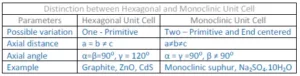(ii) Face Centred unit cell and End Centred Unit Cell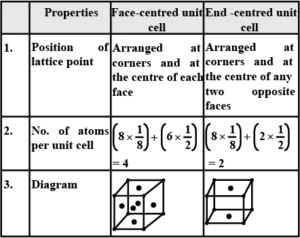Que 1.13: Explain how many portions of an atom located at (i) corner and (ii) body-centred of a cubic unit cell is part of its neighbouring unit cell.
Ans 1.13: (i) An atom located at the corner of a cubic unit cell is shared by eight adjacent unit cells. Therefore, the portion of the atom is shared by one unit cell.
(ii) An atom located at the body centre of a cubic unit cell is not shared by its neighbouring unit cell. Therefore, the atom belongs only to the unit cell in which it is present i.e., its contribution to the unit cell is 1.

Que 1.14: What is the two-dimensional coordination number of a molecule in a square close-packed layer?
Ans 1.14: In a square close-packed layer, a molecule is in contact with four of its neighbours. Therefore, the two-dimensional coordination number of a molecule in a square close-packed layer is 4.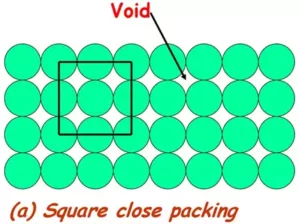Que 1.15: A compound forms a hexagonal close-packed structure. What is the total number of voids in 0.5 mol of it? How many of these are tetrahedral voids?
Ans 1.15: Number of close-packed particles = 0.5 × 6.022 × 1023 = 3.011 × 1023
Therefore, the number of octahedral voids = 3.011 × 1023
And, number of tetrahedral voids = 2 × 3.011 × 1023 = 6.022 ×1023
Therefore, total number of voids = 3.011 × 1023 + 6.022 × 1023 = 9.033 × 1023

Class 12 Solid State NCERT Solutions

Que 1.16: A compound is formed by two elements M and N. The element N forms ccp and atoms of M occupy 1/3rd of tetrahedral voids. What is the formula of the compound?
Ans 1.16: Let the number of  atoms of the element N = n
Number of tetrahedral voids = 2 × number of atoms in close packaging
Therefore
Number of tetrahedral voids = 2n
It is given that the atoms of element M occupy 1/3rd of tetrahedral voids.
So that the number of atoms of M is = (1/3) × 2n =(2/3)n
The ratio of the number of atoms of M and N
M: N = M2/3N1
= M: N = 2:3 Hence, the formula of the compound is M2N3.

Question 1.17: Which of the following lattices has the highest packing efficiency (i) simple cubic (ii) body-centred cubic and (iii) hexagonal close-packed lattice?
Ans 1.17: Hexagonal close-packed lattice has the highest packing efficiency of 74%. The packing efficiencies of simple cubic and body-centred cubic lattices are 52.4% and 68% respectively.

Que1.18: An element with a molar mass 2.7 × 10-2 kg mol-1 forms a cubic unit cell with an edge length of 405 pm. If its density is 2.7 × 103 kg m−3, what is the nature of the cubic unit cell?
Ans 1.18: It is given that density of the element, d = 2.7 × 103 kg m−3
Molar mass, M = 2.7 × 10−2 kg mol−1
Edge length, a = 405 pm = 405 × 10−12 m = 4.05 × 10−10 m
It is known that Avogadro’s number, NA = 6.022 × 1023 mol−1
Applying the relation,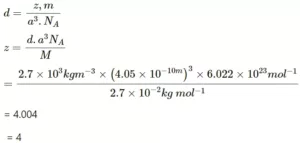This implies that four atoms of the element are present per unit cell. Hence, the unit cell is face-centred cubic (fcc) or cubic close-packed (ccp).

Que 1.19: What type of defect can arise when a solid is heated? Which physical property is affected by it and in what way?
Ans 1.19: When a solid is heated, a vacancy defect can arise. A solid crystal is said to have a vacancy defect when some of the lattice sites are vacant. Vacancy defect leads to a decrease in the density of the solid.

Que 1.20: What type of stoichiometric defect is shown by:
(i) ZnS                         (ii) AgBr
Ans 1.20: (i) ZnS shows Frenkel defect.
(ii) AgBr shows Frenkel defect as well as Schottky defect.

Class 12 Solid State NCERT Solutions

Que 1.21: Explain how vacancies are introduced in an ionic solid when a cation of higher valence is added as an impurity in it.
Ans 1.21: When a cation of higher valence is added to an ionic solid as an impurity to it, the cation of higher valence replaces more than one cation of lower valence so as to keep the crystal electrically neutral. As a result, some sites become vacant. For example, when Sr2+ is added to NaCl, each Sr2+ ion replaces two Na+ ions. However, one Sr2+ ion occupies the site of one Na+ ion and the other site remains vacant. Hence, vacancies are introduced.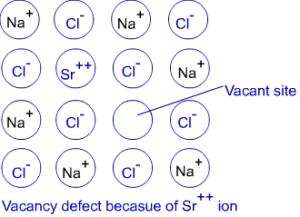Que 1.22: Ionic solids, which have anionic vacancies due to metal excess defect, develop colour. Explain with the help of a suitable example.
Ans 1.22: The colour develops because of the presence of electrons in the anionic sites. These electrons absorb energy from the visible part of radiation and get excited. For example, when crystals of NaCl are heated in an atmosphere of sodium vapours, the sodium atoms get deposited on the surface of the crystal and the chloride ions from the crystal diffuse to the surface to form NaCl with the deposited Na atoms. During this process, the Na atoms on the surface lose electrons to form Na+ ions and the released electrons diffuse into the crystal to occupy the vacant anionic sites. These electrons get excited by absorbing energy from the visible light and impart a yellow colour to the crystals.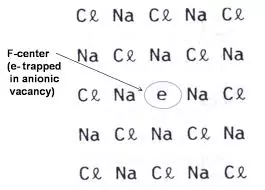Que 1.23: A group 14 element is to be converted into an n-type semiconductor by doping it with a suitable impurity. To which group should this impurity belong?
Ans 1.23: An n-type semiconductor conducts because of the presence of extra electrons. Therefore, a group 14 element can be converted to an n-type semiconductor by doping it with a group 15 element.

Que 1.24: What type of substances would make better permanent magnets, ferromagnetic or ferrimagnetic. Justify your answer.
Ans 1.24. Ferromagnetic substances would make better permanent magnets.
In a solid-state, the metal ions of ferromagnetic substances are grouped together into small regions. These regions are called domains and each domain acts as a tiny magnet. In an unmagnetised piece of a ferromagnetic substance, the domains are randomly oriented. As a result, the magnetic moments of the domains get cancelled. However, when the substance is placed in a magnetic field, all the domains get oriented in the direction of the magnetic field and a strong magnetic effect is produced. The ordering of the domains persists even after the removal of the magnetic field. Thus, the ferromagnetic substance becomes a permanent magnet.

Class 12 Solid State NCERT Solutions

Exercise Solutions
The Solid State

Que 1. Define the term ‘amorphous’. Give a few examples of amorphous solids.
Ans 1:
A solid is said to be amorphous if the constituent particles are not arranged in any regular fashion. They may have only short-range order. Amorphous solids are generally obtained when the melts are rapidly cooled, e.g., glass, plastics, amorphous silica, etc.

Que 2. What makes a glass different from a solid such as quartz? Under what conditions could quartz be converted into glass
Ans 2: Glass is an amorphous solid in which the constituent particles (SiO4 tetrahedral) have only a short-range order and there is no long-range order. In quartz, the constituent particles (SiO4 tetrahedral) have both short-range as well as long-range orders. On melting quartz and then cooling it rapidly, it is converted into glass.

Que 3: Classify each of the following solids as ionic, metallic, molecular, network (covalent) or amorphous. Tetraphosphorus decoxide, P4O10, Ammonium phosphate, (NH4)3PO4, SiC, l2, P4, Plastic, Graphite, Brass, Rb, LiBr, Si.
Ans 3: P4O10 – molecular, (NH4)3PO4 – ionic, SiC – network (covalent), I2 – molecular, P4 – molecular, plastic – amorphous, graphite – covalent, brass – metallic, Rb – metallic, LiBr – ionic, Si – covalent

Que 4. (i) What is meant by the term ‘coordination number’?
(ii) What is the coordination number of atoms:
(a) in a cubic close-packed structure?
(b) in a body-centred cubic structure?
Ans 4: (i)
Coordination number is defined as the number of nearest neighbours in a close packing. In ionic crystals, the coordination number of an ion in the crystal is the number of oppositely charged ions surrounding that particular ion.

(ii) (a) Coordination number of atoms in a cubic close-packed structure is 12.
(b) Coordination number of atoms in a body-centred cubic structure is 8.

Que 5. How can you determine the atomic mass of an unknown metal if you know its density and the dimension of its unit cell? Explain.
Ans 5.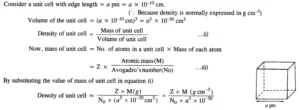Class 12 Solid State NCERT Solutions

Que 6. The stability of a crystal is reflected in the magnitude of its melting point. Comment. Collect melting points of solid water, ethyl alcohol, diethyl ether and methane from a data book. What can you say about the intermolecular forces between these molecules?
Ans 6.
The melting points of some compounds are given below

Water = 273 K, Ethyl alcohol = 155.7 K, Diethyl ether = 156.8 K, Methane = 90.5 K.
The higher the melting point, the stronger are the forces holding the constituent particles together and hence greater is the stability.
The intermolecular forces in water and ethyl alcohol are mainly hydrogen bonding. The higher melting point of water as compared to alcohol shows that hydrogen bonding in ethyl alcohol molecules is not as strong as in water molecules. Diethyl ether is a polar molecule. The intermolecular forces present in them are the dipole-dipole attraction. Methane is a non-polar molecule. The only forces present in them are the weak van der Waals forces (London or Dispersion Forces).

Que 7. How will you distinguish between the following pairs of terms?
(a) Hexagonal close-packing and cubic close-packing
(b) Crystal lattice and unit cells
(c) Tetrahedral void and octahedral void
Ans 7. (a) In Hexagonal Close Packing (hcp), the spheres of the third layer are vertically above the spheres of the first layer (ABABAB….type). On the other hand, in cubic close packing (ccp), the spheres of the fourth layer are present above the spheres of the first layer (ABCABC…..type).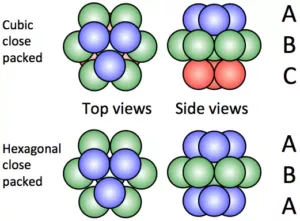(b) Crystal lattice: It depicts the actual shape as well as the size of the constituent particles in the crystal. It is, therefore, called space lattice or crystal lattice.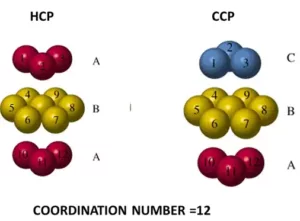Unit cell: Each brick represents the unit cell while the block is similar to space or crystal lattice. Thus, a unit cell is the fundamental building block of the space lattice.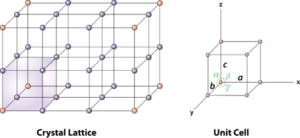(c) Tetrahedral void: A tetrahedral void is formed when a triangular void made by three spheres of a particular layer and touching each other.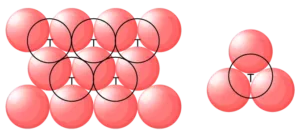Octahedral void: An octahedral void or site is formed when three spheres arranged at the corners of an equilateral triangle are placed over another set of spheres.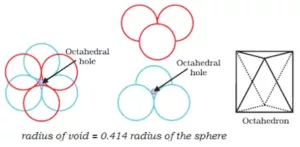Que 8. How many lattice points are there is one unit cell of each of the following lattices?
(i) Face centred cubic
(if) Face centred tetragonal
(iii) Body Centred Cubic
Ans 8. (i) There are 14 (8 from the corners + 6 from the faces) lattice points in face-centred cubic.
(ii) There are 14 (8 from the corners + 6 from the faces) lattice points in face-centred tetragonal.
(iii) There are 9 (1 from the centre + 8 from the corners) lattice points in body-centred cubic.

Que 9. Explain
(i) The basis of similarities and differences between metallic and ionic crystals.
(ii) Ionic solids are hard and brittle.
Ans 9. (i) Metallic and ionic crystals
(a) There is an electrostatic force of attraction in both metallic and ionic crystals.
(b) Both have high melting points.
(c) Bonds are non-directional in both cases.
Differences:
(a) Ionic crystals are bad conductors of electricity in the solids state as ions are not free to move. They can conduct electricity only in a die molten state or in an aqueous solution. Metallic crystals are good conductors of electricity in the solid-state as electrons are free to move.
(b) Ionic bond is strong due to strong electrostatic forces of attraction.
The metallic bond may be strong or weak depending upon the number of valence electrons and the size of the kernels.
(ii) Ionic solids are hard and brittle. Ionic solids are hard due to the presence of strong electrostatic forces of attraction. The brittleness in ionic crystals is due to the non-directional bonds in them.

Que 10. Calculate the efficiency of packing in the case of a metal crystal for
(i) Simple Cubic
Answer: Let the side of a simple cubic lattice is ‘a’ and the radius of the atom present in it is ‘r’.
Since, edges of atoms touch each other, therefore, a = 2r (for simple cubic lattice)
Volume of cube =Side3 = a3 = (2r)3
The volume of one atom =4/3πr3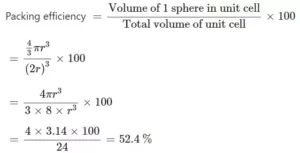(ii) Body-Centred Cubic: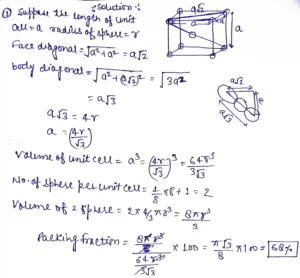Class 12 Solid State NCERT Solutions

Que 11. Silver crystallises in fcc lattice. If the edge length of the cell is 4.07 x 10-8 cm and density is 10.5 g cm-3, calculate the atomic mass of silver.
Ans 11. It is given that the edge length, a = 4.077 × 10−8 cm
Density, d = 10.5 g cm−3
As the lattice is fcc type, the number of atoms per unit cell, z = 4
We also know that NA  = 6.022 × 1023 mol−1
Using the relation: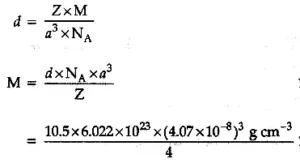= 107.09 g/mol

Que 12. A cubic solid is made of two elements P and Q. Atoms of Q are at the corners of the cube and P at the body-centre. What is the formula of the compound? What is the coordination number of P and Q?
Ans 12. It is given that the atoms of Q are present at the corners of the cube.
Therefore, the number of atoms of Q in one unit cell = 8 x (1/8) = 1
It is also given that the atoms of P are present at the body-centre.
Therefore, the number of atoms of P in one unit cell = 1
This means that the ratio of the number of P atoms to the number of Q atoms, P:Q = 1:1
Hence, the formula of the compound is PQ.
The coordination number of both P and Q is 8.

Que 13. Niobium crystallises in a body-centred cubic structure. If the density is 8.55 g cm–3, calculate the atomic radius of niobium using its atomic mass of 93 u.
Ans 13. Given, density (d) = 8.55 g cm-3
Atomic Mass (M) = 93 u = 93 g mol-1
We know, Avogadro Number NA = 6.022 × 1023mol-1
Since the given lattice is bcc
Hence, the number of atoms per unit cell (z) = 2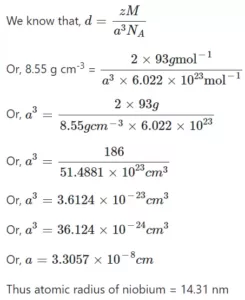Que 14. If the radius of the octahedral void is r and the radius of the atoms in close-packing is R, derive the relation between rand R.
Ans 14.  A sphere is fitted into the octahedral void as shown in the diagram.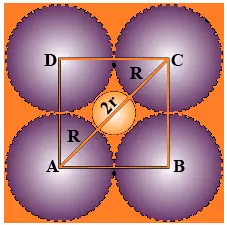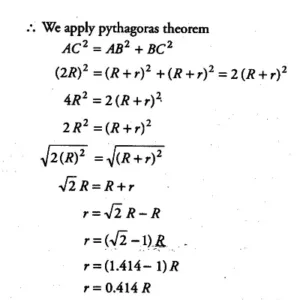Que 15. Copper crystallises into a fee lattice with an edge length of 3.61 x 10-8 cm. Show that the calculated density is in agreement with its measured value of 8.92 g/cm3.
Ans 15. Given, edge length (a) = 3.61 × 10-8cm
Hence, the number of atoms per unit cell in fcc (z) = 4
Avogadro’s Number (NA) = 6.022 ××1023 g mol-1
To prove, density (d) = 8.92 g cm-3
We know that density (d) = zM/a3NA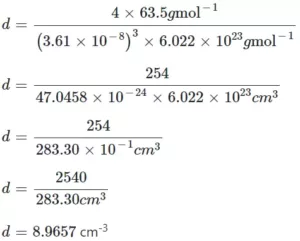Class 12 Solid State NCERT Solutions

Que 16. The analysis shows that nickel oxide has the formula Ni0.98 O1.00. What fractions of nickel exist as Ni2+ and Ni3+ ions?
Ans 16. Given, the formula of nickel oxide = Ni0.98O1.00
So, Ni : O = 0.98 : 1.00 = 98 : 100
Total charge on O2- = 100 × (-2) = -200
Now, let number of Ni2+ = x
So, number of Ni3+ = 98 – x
Since the compound is neutral
Hence, number of Ni2+ ions + number of Ni3+ ions + number of O2- ions = 0
= x(−2) + (98−x) × (−3) + (−200) = 0
= −2x – 294 + 3x – 200 = 0
= x – 94 = 0
x = 94
So, number of Ni2+ ions = 94
Number of Ni3+ ions = 98 – 94 = 4
Now, fraction of nickel existing as Ni2+ ions = 94/98 = 0.959
Fraction of nickel existing as Ni3+ = 4/98 = 0.041

Que 17. What are semi-conductors? Describe the two main types of semiconductors and contrast their conduction mechanisms.
Ans 17. Semiconductor: – Solids having an intermediate range of conductivity, i.e. from 10–6 to 10ohm–1 m–1 are called semiconductors. Semiconductors are of the following two types:
(a) n-type of semiconductors
(b) p-type of semiconductors
(a) n-type semiconductors – An n-type semiconductor is an intrinsic semiconductor doped with phosphorus (P), arsenic (As), or antimony (Sb) as an impurity. Silicon of Group IV has four valence electrons and phosphorus of Group V has five valence electrons. If a small amount of phosphorus is added to a pure silicon crystal, one of the valence electrons of phosphorus becomes free to move around (free-electron) as a surplus electron. When this free electron is attracted to the “+” electrode and moves, the current flows.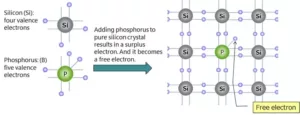(b) p – type of semiconductors – The extrinsic pType Semiconductor is formed when a trivalent impurity is added to a pure semiconductor in a small amount, and as a result, a large number of holes are created in it. A large number of holes are provided in the semiconductor material by the addition of trivalent impurities like Gallium and Indium.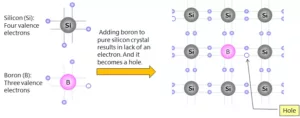Que 18. Non-stoichiometric cuprous oxide, Cu2O can be prepared in the laboratory. In this oxide, the copper to oxygen ratio is slightly less than 2:1. Can you account for the fact that this substance is a p-type semiconductor?
Ans 18. When cuprous oxide is prepared in the laboratory; the ratio of copper to the oxygen in the compound becomes slightly less than 2:1. This happens because some of the Cu+ ions are replaced by the Cu2+ ions. In this process, one Cu2+ ion replaces two Cu+ ions. As two Cu+ ions are replaced by one Cu2+ ion, this creates defects because of creating vacant space, i.e. positive holes.
Because of the creation of holes due to this defect; this compound conducts electricity through these positive holes.
As semiconductors that are formed by electron-deficient impurities are called p-type semiconductors; thus, cuprous oxides so formed in the laboratory are the p-type of semiconductors.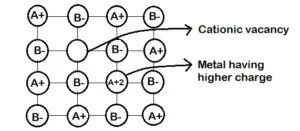Que 19. Ferric oxide crystallises in a hexagonal dose- packed array of oxide ions with two out of every three octahedral holes occupied by ferric ions. Derive the formula of the ferric oxide.
Ans 19. Given, Ferric oxide crystallizes in a hexagonal close-packed array of oxide ions.
Two out of every three octahedral holes are occupied by ferric ions.
Let the number of oxide ions = x
Therefore the number of octahedral voids = x
Since, two out of every three octahedral holes are filled by ferric ions,
Thus, voids filled by ferric ions
Fe3+ = 2/3x
Therefore, the number of ferric ions
Fe3+ = 2/3x
Now, the ratio of ferric ions to the oxide ions
i.e. Fe3+ : O2- =2/3x : x
= 2 : 3  Hence, the formula of ferric oxide is Fe2O3

Que 20. Classify each of the following as being either a p-type or n-type semiconductor:
i). Ge doped with In
ii). B doped with Si.
Ans 20. i). Ge is a group 14 element and In is a group 13 element. Hence, an electron-deficient hole is created and therefore, it is a p-type semiconductor.
ii). Boron is a group 13 element and Si is a group 14 element, there will be a free electron, So, it is an n-type semiconductor.

Que 21. Gold (atomic radius = 0.144 nm) crystallises in a face-centred unit cell. What is the length of the side of the unit cell?
Ans 21. Given, atomic radius = 0.144 nm
Type of unit cell = face centred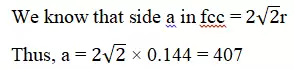Thus side of the given cell = 407 nm

Que 22. In terms of band theory, what is the difference
(i) Between a conductor and an insulator
(ii) Between a conductor and a semiconductor?
Ans 22. In most of the solids and in many insulating solids conduction takes place due to the migration of electrons under the influence of the electric field. However, in ionic solids, it is the ions that are responsible for conducting behaviour due to their movement.
(i) Between a conductor and an insulator: In conductors there is no energy gap between the valence band, which facilitates the flow of electrons easily under an applied electric field and metals show conductivity. While in insulators there is a large energy gap between the valence band and electrons cannot jump to it i.e. large energy gap prevents the flow of electricity.
(ii) Between a conductor and a semiconductor: In conductors there is no energy gap between the valence band and conduction band, which facilitates the flow of electrons easily under an applied electric field and metals show conductivity.
While in semiconductors, there is a small energy gap between the valence bond and the conduction band. The small gap between bands facilitates some electrons to jump to the conduction band by acquiring extra energy.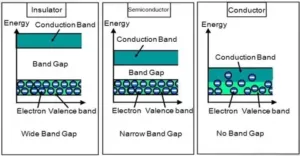Que 23. Explain the following terms with suitable examples :
(i) Schottky defect
(ii) Frenkel defect
(iii) Interstitial defect
(iv) F-centres
Ans 23. (i) Schottky defect: Schottky defect is basically a vacancy defect. In this defect, an equal number of cations and anions are missing to maintain electrical neutrality. It decreases the density of a substance. It is a common defect in ionic compounds of high coordination number where both cations and anions are of the same size, e.g., KCl, NaCl, KBr, etc.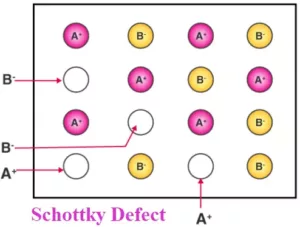(ii) Frenkel defect:  This defect arises when some of the ions (generally cation due to its small size) in the lattice occupy interstitial sites leaving lattice sites vacant. This defect is generally found in ionic crystals where anion is much larger in size than the cation, e.g., AgBr, ZnS, etc. Due to this defect density does not change.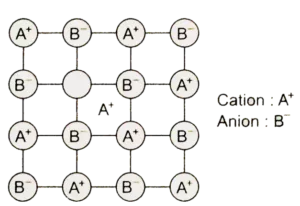(iii) Interstitial defect: Sometime in the formation of lattice structure some of the atoms or ions occupy the vacant interstitial site, and are known as interstitials. These interstitials are generally small size non-metals, such as H, B, C, etc. The defect that arises because of interstitials is called an interstitial defect.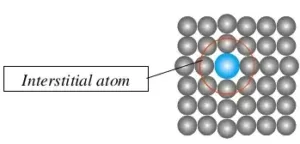(iv) F-centres: This is a type of defect and called a metal excess defect. These type of defects seen because of the missing of anions from the regular site leaving a hole which is occupied by an electron to maintain the neutrality of the compound. The hole occupied by the electron is called F-centre and responsible for showing colour by the compound.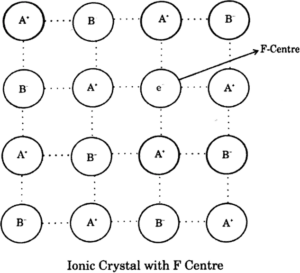Que 24. Aluminium crystallises in a cubic close-packed structure. Its metallic radius is 125 pm.
(i) What is the length of the side of the unit cell?
(ii) How many unit cells are there in 1.00 cm3 of aluminium?
Ans 24. Given, the radius of an atom (r) = 125 pm
(i) For ccp structure, we know that
Where, r = radius and a = length of side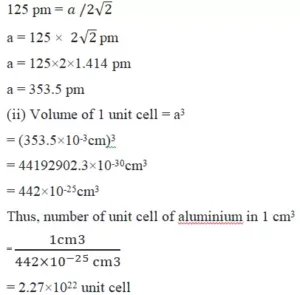Class 12 Solid State NCERT Solutions

Que 25. If NaCI is doped with 10-3 mol % SrCl2, what is the concentration of cation vacancies?
Ans 25. Let moles of NaCI = 100
∴ Moles of SrCl2 doped = 10-3
Each Sr2+ will replace two Na+ ions. To maintain electrical neutrality it occupies one position and thus creates one cation vacancy.
∴ Moles of cation vacancy in 100 moles NaCI = 10-3
Moles of cation vacancy in one mole
NaCI = 10-3 × 10-2 = 10-5
∴ Number of cation vacancies
= 10-5 × 6.022 × 1023 = 6.022 × 1018 mol-1.

Que 26. Explain the following with suitable example:
(i) Ferromagnetism
(ii) Paramagnetism
(iii) Ferrimagnetism
(iv) Antiferromagnetism
(v) 12-16 and 13-15 group compounds.
Ans 26. (i) Ferromagnetism: Substances that are attracted strongly with magnetic field are called ferromagnetic substances, such as cobalt, nickel, iron, gadolinium, chromium oxide, etc. Ferromagnetic substances can be permanently magnetized also. In a solid-state, the metal ions of ferromagnetic substances are grouped together into small regions called domains and each domain acts as a tiny magnet.
(ii) Paramagnetism: Substances that are attracted slightly by the magnetic field and do not retain the magnetic property after removal of the magnetic field is called paramagnetic substances. They are magnetized in the same direction as that of the applied field. This property is shown by those substances whose atoms, ions or molecules contain unpaired electrons For example O2-, Cu2+, Fe3+, Cr3+, Magnesium, molybdenum. Substances that are slightly attracted in the magnetic field and in which domains are grouped in parallel and anti-parallel direction but in unequal number are called ferromagnetic substances and this property is called ferrimagnetism. Examples include Fe3O4 (magnetite), ferrites such as MgFe2O4 and ZnFe2O4.
(iv) Antiferromagnetism: Substances in which domain structure is similar to ferromagnetic substances but are oriented oppositely, which cancel the magnetic property are called antiferromagnetic substances and this property is called antiferromagnetism. For example; MnO.(v) 12-16 and 13-15 group compounds: When the solid-state materials are produced by the combination of elements of groups 13 and 15, the compounds thus obtained are called 13-15 compounds. For example, InSb, AlP, GaAs, etc.

Combination of elements of groups 12 and 16 yields some solid compounds which are referred as 12-16 compounds. For example, ZnS, CdS, CdSe, HgTe, etc. In these compounds, the bonds have an ionic character.

Class 12 Solid State NCERT Solutions